For those wishing to follow along with the R-based demo in class, click here for the companion R script for this lecture.

Now we will run the same titanic analysis, but using a machine learning method- in this case, Random Forest. Just for kicks!

Let’s set up the workspace with the functions and packages we need!

suppressMessages(suppressWarnings(library(party)))

source_github <- function(baseurl,scriptname) {
suppressMessages(suppressWarnings(require(RCurl)))

# read script lines from website
url <- sprintf("%s%s",baseurl,scriptname)
script <- getURL(url, ssl.verifypeer = FALSE)

script <- gsub("\r\n", "\n", script)     # get rid of carriage returns (not sure why this is necessary...)

# parse lines and evaluate in the global environement
eval(parse(text = script), envir= .GlobalEnv)
}

baseurl = "https://raw.githubusercontent.com/kevintshoemaker/Random-Forest-Functions/master/"
source_github(baseurl,"RF_Extensions.R")

First, we read in the data

titanic <- read.csv("titanic.csv",header=T)
head(titanic)
##   PassengerId Survived Pclass
## 1           1        0      3
## 2           2        1      1
## 3           3        1      3
## 4           4        1      1
## 5           5        0      3
## 6           6        0      3
##                                                  Name    Sex Age SibSp
## 1                             Braund, Mr. Owen Harris   male  22     1
## 2 Cumings, Mrs. John Bradley (Florence Briggs Thayer) female  38     1
## 3                              Heikkinen, Miss. Laina female  26     0
## 4        Futrelle, Mrs. Jacques Heath (Lily May Peel) female  35     1
## 5                            Allen, Mr. William Henry   male  35     0
## 6                                    Moran, Mr. James   male  NA     0
##   Parch           Ticket    Fare Cabin Embarked
## 1     0        A/5 21171  7.2500              S
## 2     0         PC 17599 71.2833   C85        C
## 3     0 STON/O2. 3101282  7.9250              S
## 4     0           113803 53.1000  C123        S
## 5     0           373450  8.0500              S
## 6     0           330877  8.4583              Q

When using categorical variables, we should make sure they are encoded as factors, not as numeric. Use class(data$Resp) to check the encoding, and use as.factor(data$Resp) to encode your vector as a factor.

titanic$Survived <- as.factor(titanic$Survived)

Now let’s define the predictors and response:

predictorNames <- c(  "Sex",       # nice readable names
"Age",
"Sibs/spouses",
"Parents/children",
"Fare"
)

pred.names=c(  "Sex",
"Age",
"SibSp",
"Parch",
"Fare"
)
# cbind(pred.names,predictorNames)

response="Survived"

formula1 <- as.formula(paste(response,"~",paste(pred.names,collapse="+")))    # formula for the RF model

## Run a conditional inference tree

This is also known as a CART analysis- single tree!

TerrMamm.tr <- ctree(formula=formula1, data=titanic, controls = ctree_control(mincriterion = 0.85,maxdepth = 3))

plot(TerrMamm.tr)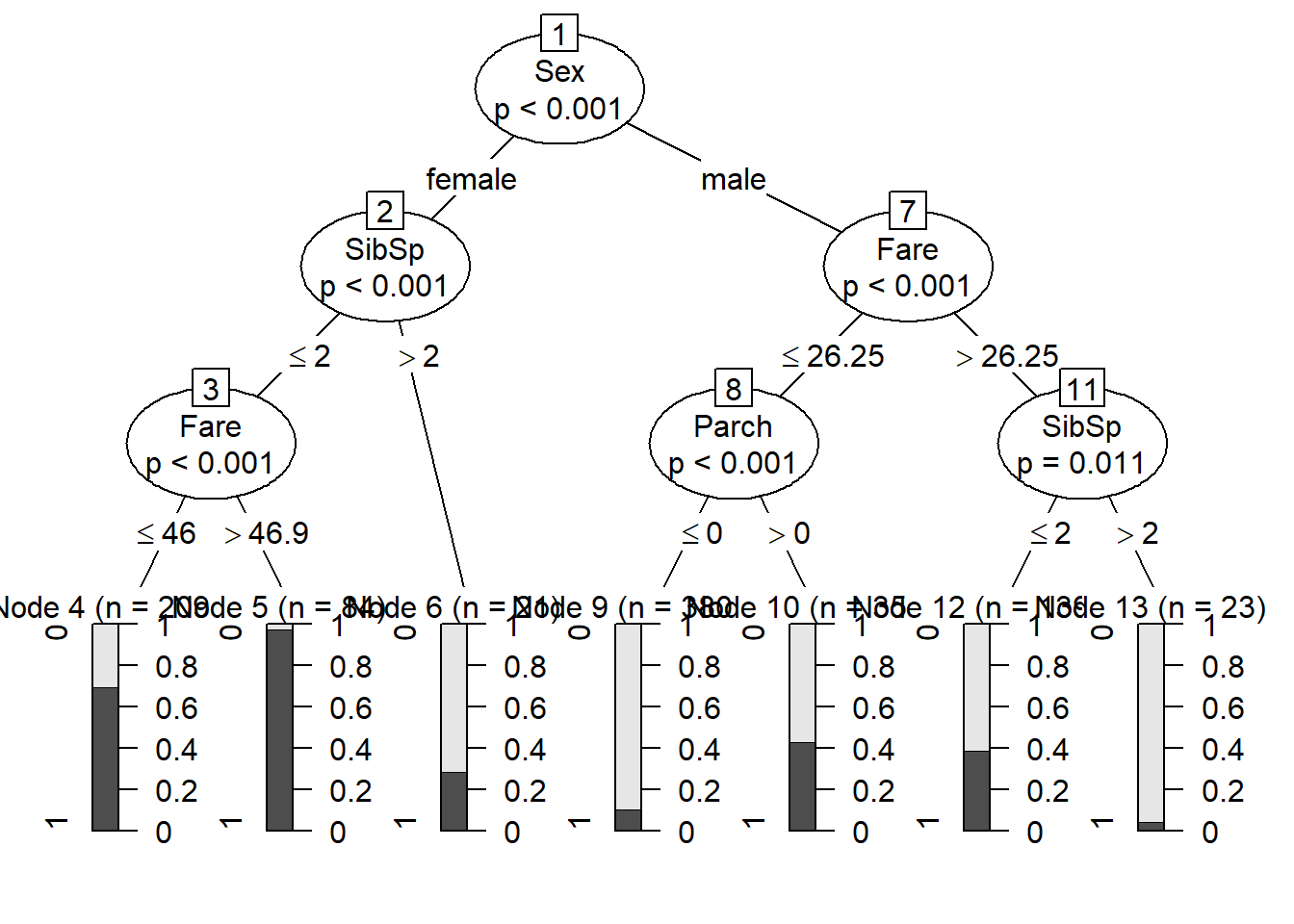But remember that a single tree is not very robust- these are very liable to over-fitting! Random forest gets around this using random sampling in several creative ways!

Like most machine learning algorithms, we can “tune” the algorithm in several different ways. If this were a “real” analysis, I would try several alternative tunings.

cforestControl <- cforest_unbiased(ntree=500,mtry=3)   # change back to 500!!
cforestControl@fraction <- 0.75
cforestControl@gtctrl@mincriterion <- 0.75

rf_model1 <- cforest(formula1, controls=cforestControl, data=titanic)

## Variable importance

One thing we can easily get from a RF analysis is an index of the relative importance of each predictor variable

    # get the importance values
model1_importance<-varimp((rf_model1), conditional= FALSE)

lengthndx <- length(model1_importance)
#par(mai=c(0.95,3.1,0.6,0.4))
par(mai=c(1.4,3.4,0.6,0.9))
col <- rainbow(lengthndx, start = 3/6, end = 4/6)
barplot(height=model1_importance[order(model1_importance,decreasing = FALSE)],
horiz=T,las=1,main="Order of Importance of Predictor Variables",
xlab="Index of overall importance",col=col,
names.arg=predictorNames[match(names(model1_importance),pred.names)][order(model1_importance,decreasing = FALSE)])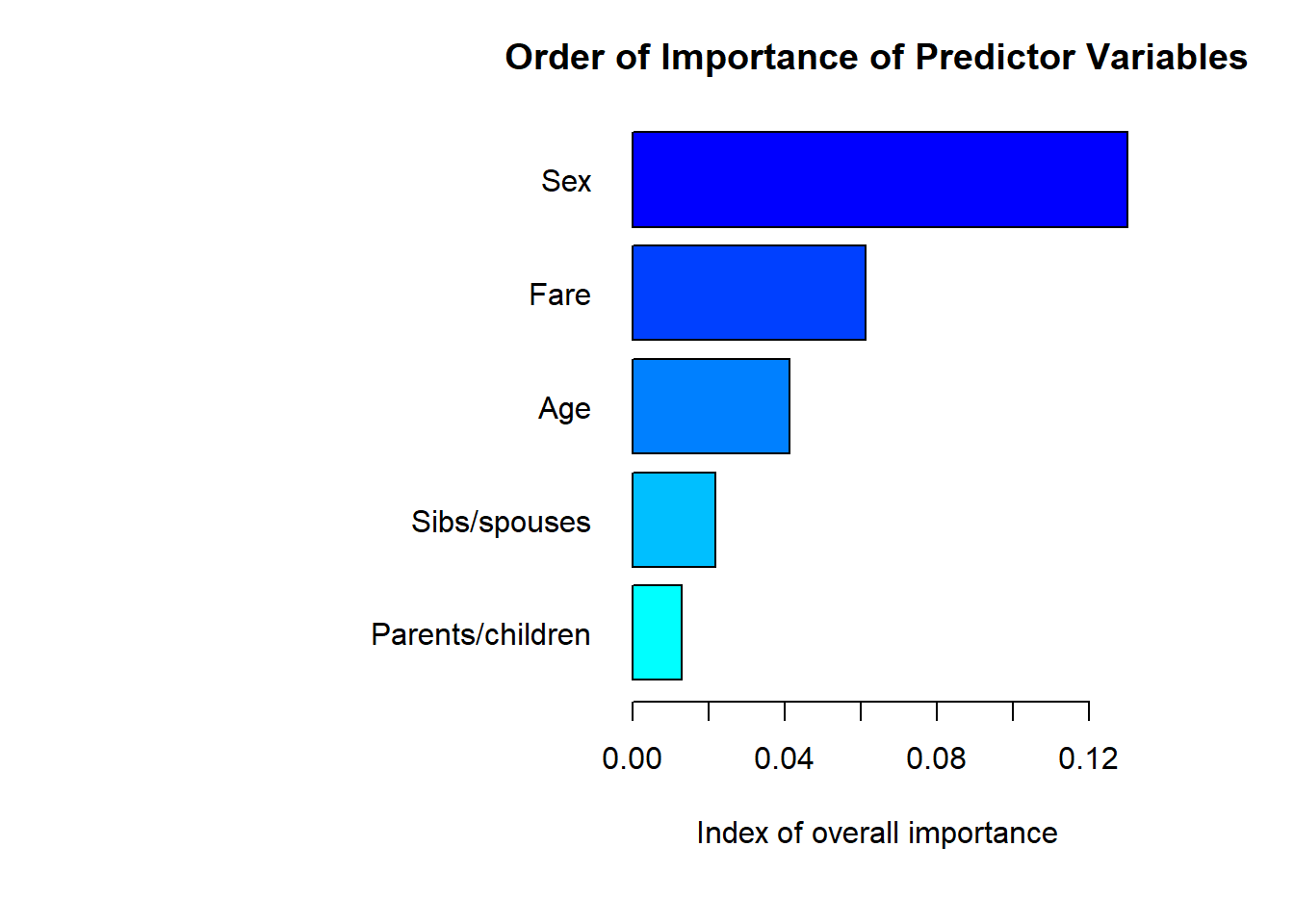## Univariate plots

We can also generate univariate plots, also known as “partial dependence plots”:

##### Make univariate plots of the relationships- plot one relationship at a time

RF_UnivariatePlots(object=rf_model1, varimp=model1_importance, data=titanic,  #
predictors=pred.names, labels=predictorNames, allpredictors=pred.names,plot.layout=c(1,1))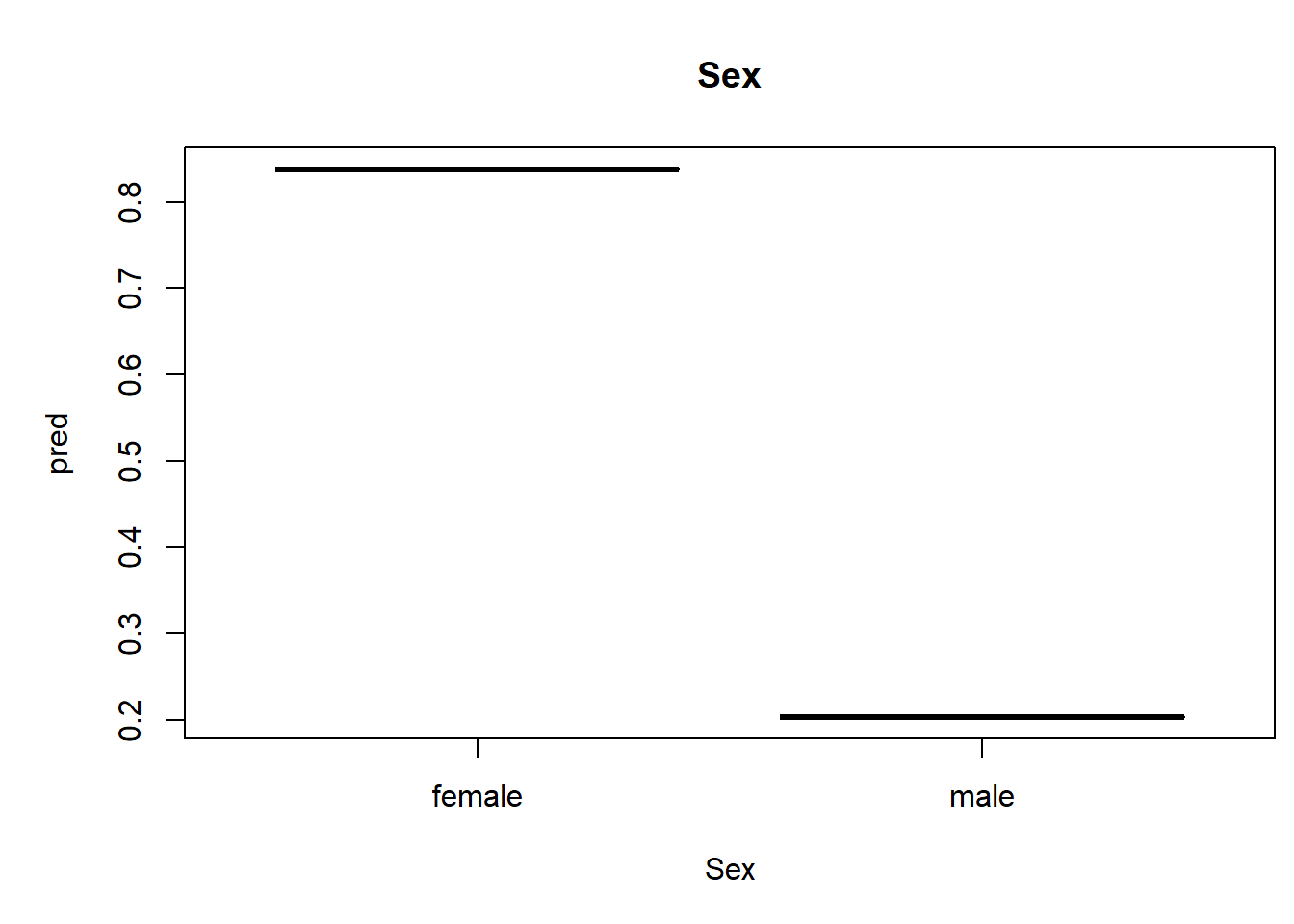RF_UnivariatePlots(object=rf_model1, varimp=model1_importance, data=titanic,  #
predictors=pred.names, labels=predictorNames, allpredictors=pred.names,plot.layout=c(1,1))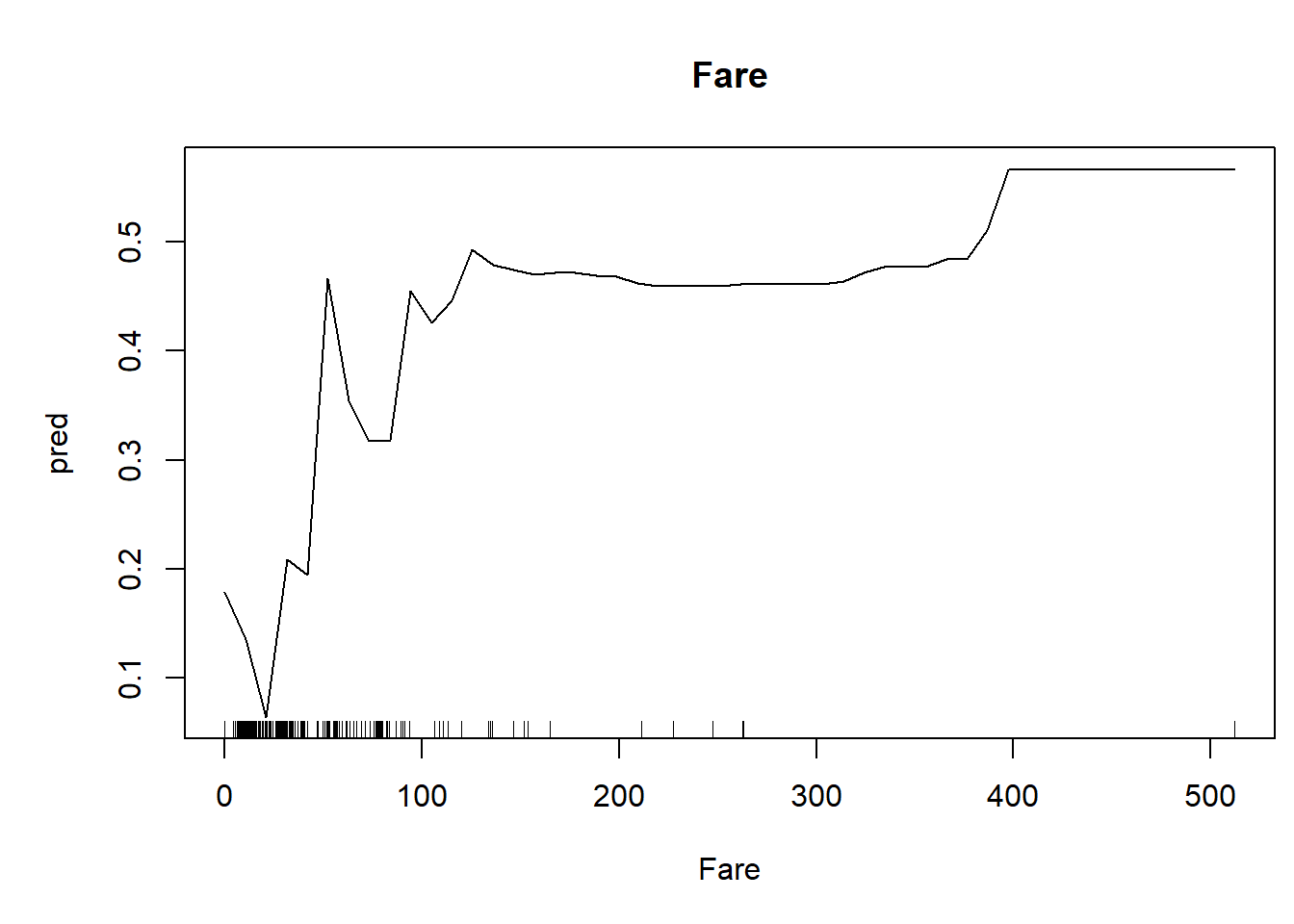RF_UnivariatePlots(object=rf_model1, varimp=model1_importance, data=titanic,  #
predictors=pred.names, labels=predictorNames, allpredictors=pred.names,plot.layout=c(1,1))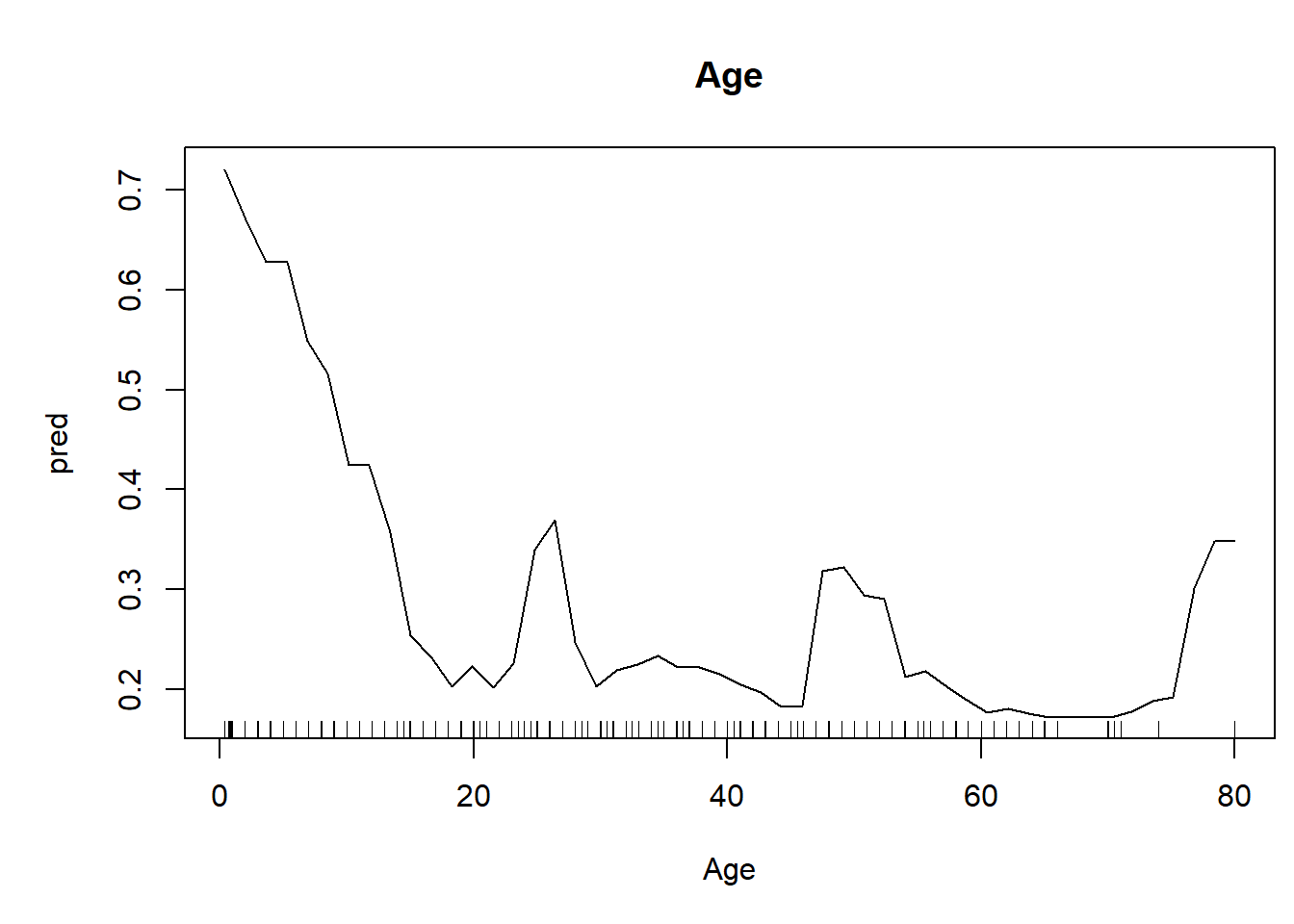RF_UnivariatePlots(object=rf_model1, varimp=model1_importance, data=titanic,  #
predictors=pred.names, labels=predictorNames, allpredictors=pred.names,plot.layout=c(1,1))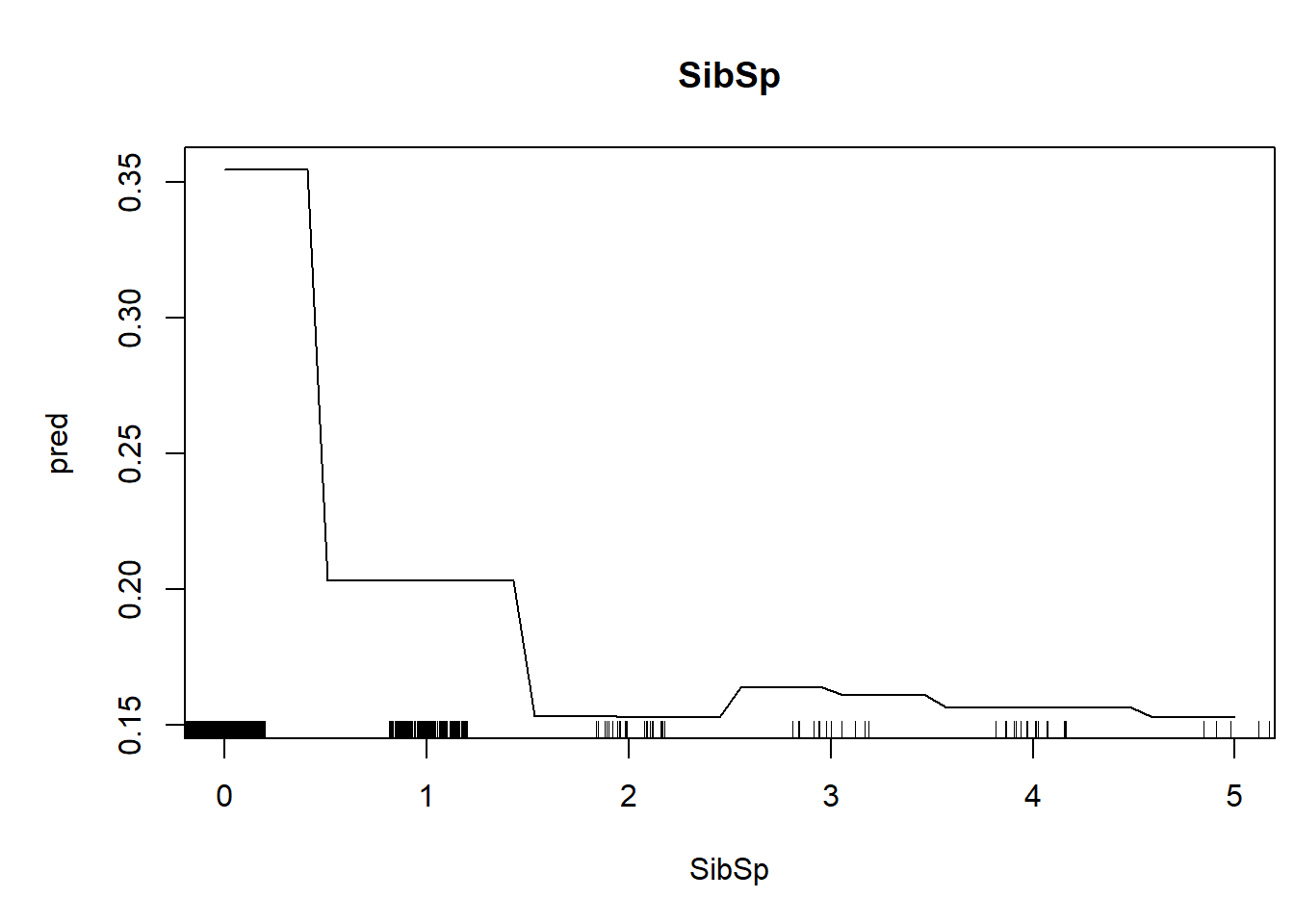Finally, and perhaps most importantly, we can find and plot the most important interactions!

# NOTE: this one can take a very long time   ...
rf_findint <- RF_FindInteractions(object=rf_model1,data=titanic,predictors=pred.names)
## 1  2  3  4
rf_findint$interactions1 ## Sex Age SibSp Parch Fare ## Sex 0 4.4622 5.8858 7.8177 1.2221 ## Age 0 0.0000 3.2911 2.1492 0.6831 ## SibSp 0 0.0000 0.0000 0.1774 1.5752 ## Parch 0 0.0000 0.0000 0.0000 1.0534 ## Fare 0 0.0000 0.0000 0.0000 0.0000 rf_findint$rank.list1
##   var1.index var1.names var2.index var2.names int.size
## 1          4      Parch          1        Sex   7.8177
## 2          3      SibSp          1        Sex   5.8858

## Interactions

### plot interaction strength

lengthndx <- min(9,nrow(rf_findint$rank.list1)) par(mai=c(0.95,3.1,0.6,0.4)) barplot(height=(rf_findint$rank.list1[c(1:min(9,nrow(rf_findint$rank.list1))),5][c(lengthndx:1)]), horiz=T,las=1,main=paste(response, sep=""), xlab="Index of interaction strength",col=brewer.pal(lengthndx,"Blues"), names.arg=paste("",predictorNames[match(rf_findint$rank.list1[,2][c(lengthndx:1)],pred.names)],"\n",predictorNames[match(rf_findint$rank.list1[,4][c(lengthndx:1)],pred.names)],sep="") ) ## Warning in brewer.pal(lengthndx, "Blues"): minimal value for n is 3, returning requested palette with 3 different levels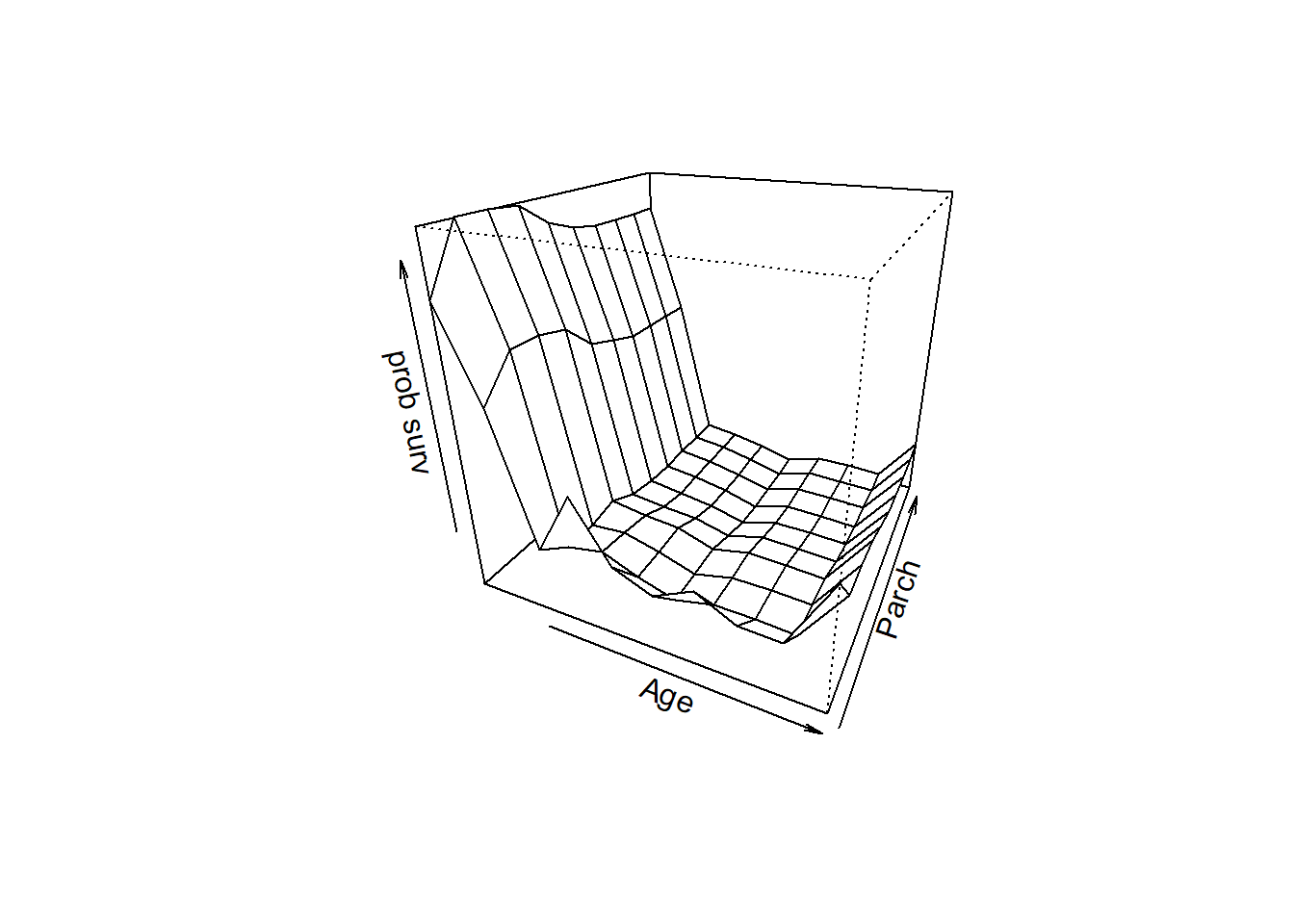Now let’s visualize the interactions: rf_findint$rank.list1
##   var1.index var1.names var2.index var2.names int.size
## 1          4      Parch          1        Sex   7.8177
## 2          3      SibSp          1        Sex   5.8858
fam="binomial"

RF_InteractionPlots(x=1,y=4,object=rf_model1,data=titanic,predictors=pred.names,family=fam) 
## maximum value =  0.88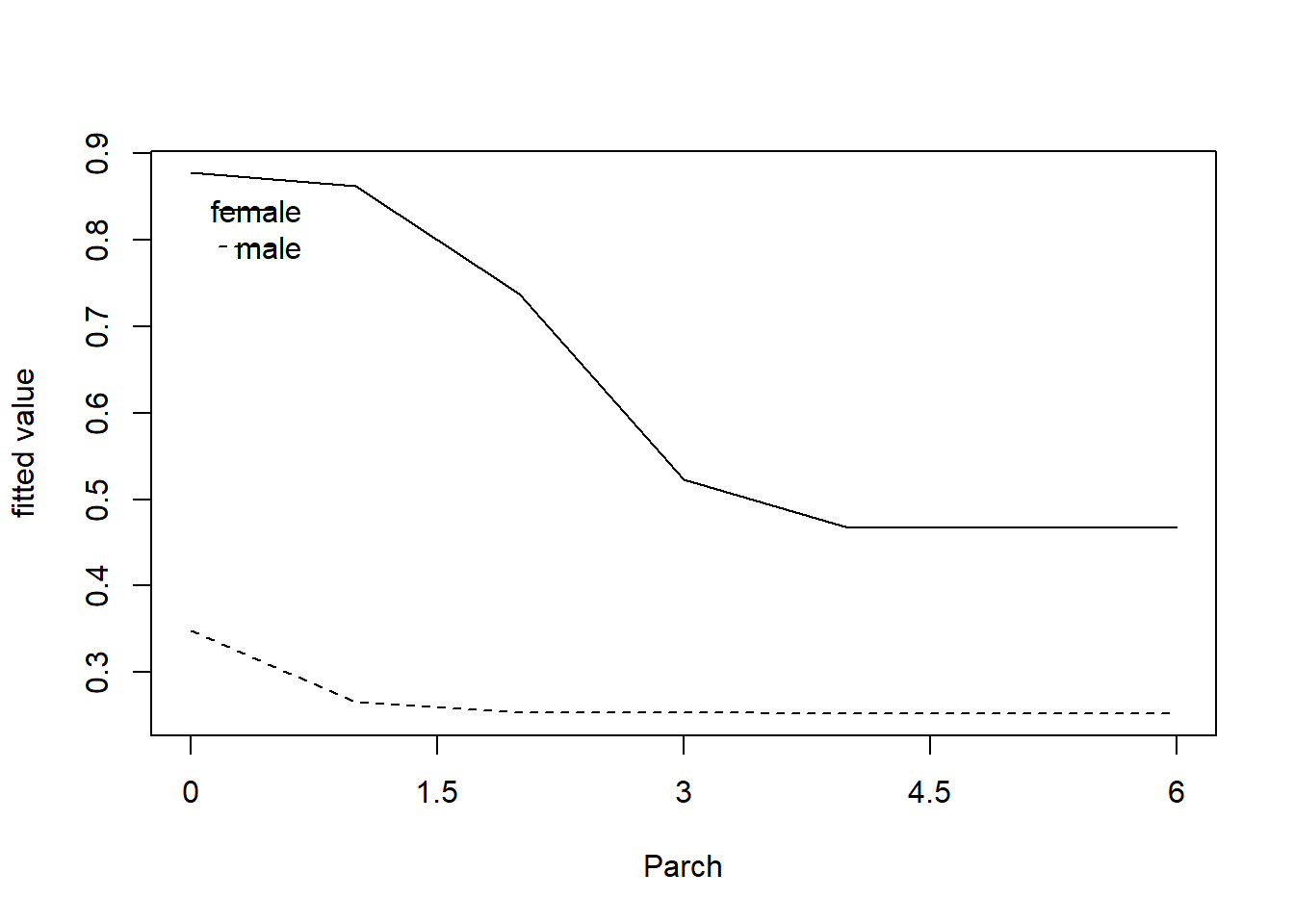RF_InteractionPlots(x=1,y=3,object=rf_model1,data=titanic,predictors=pred.names,family=fam) 
## maximum value =  0.88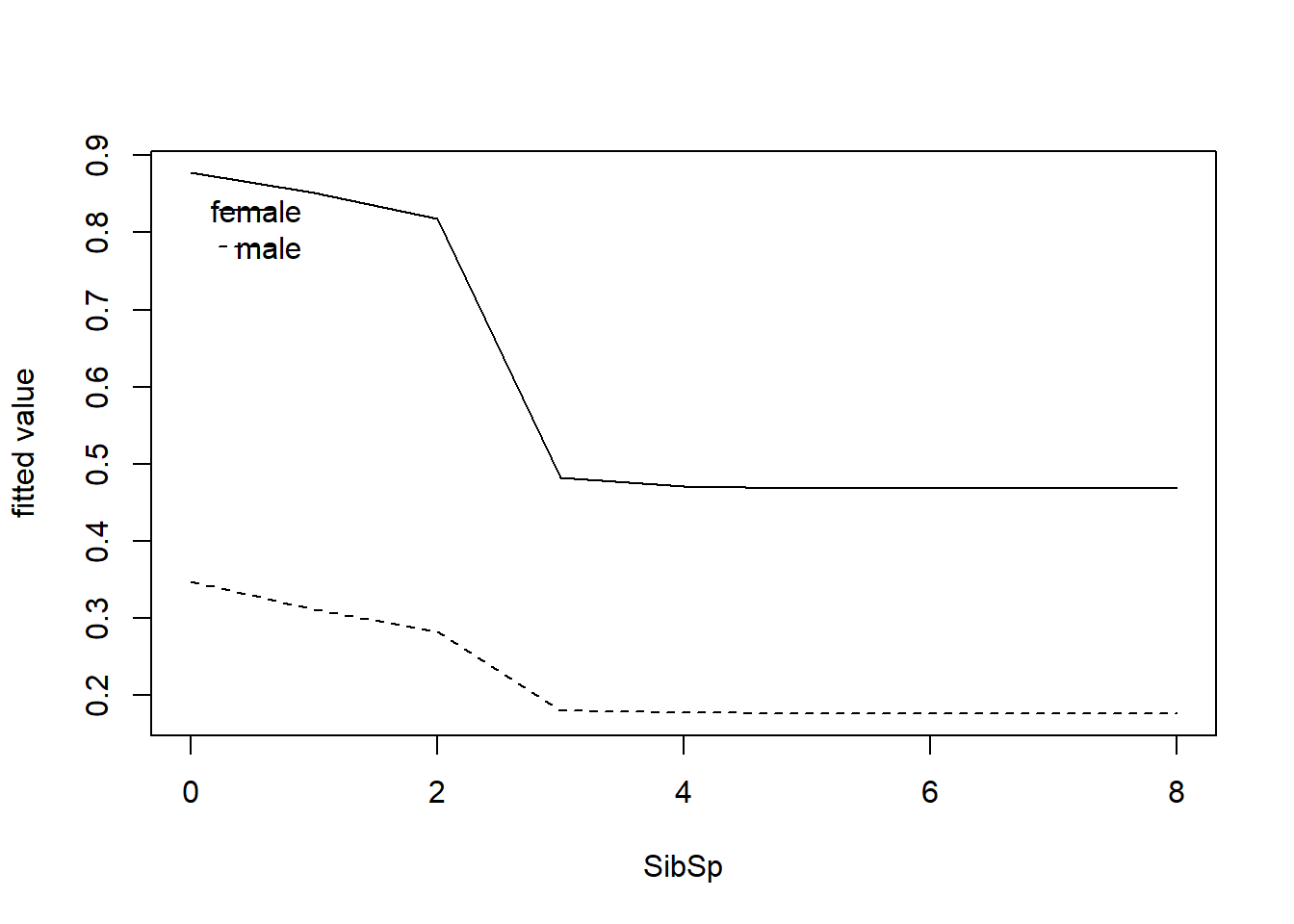RF_InteractionPlots(x=2,y=4,object=rf_model1,data=titanic,predictors=pred.names,family=fam) 
## maximum value =  0.72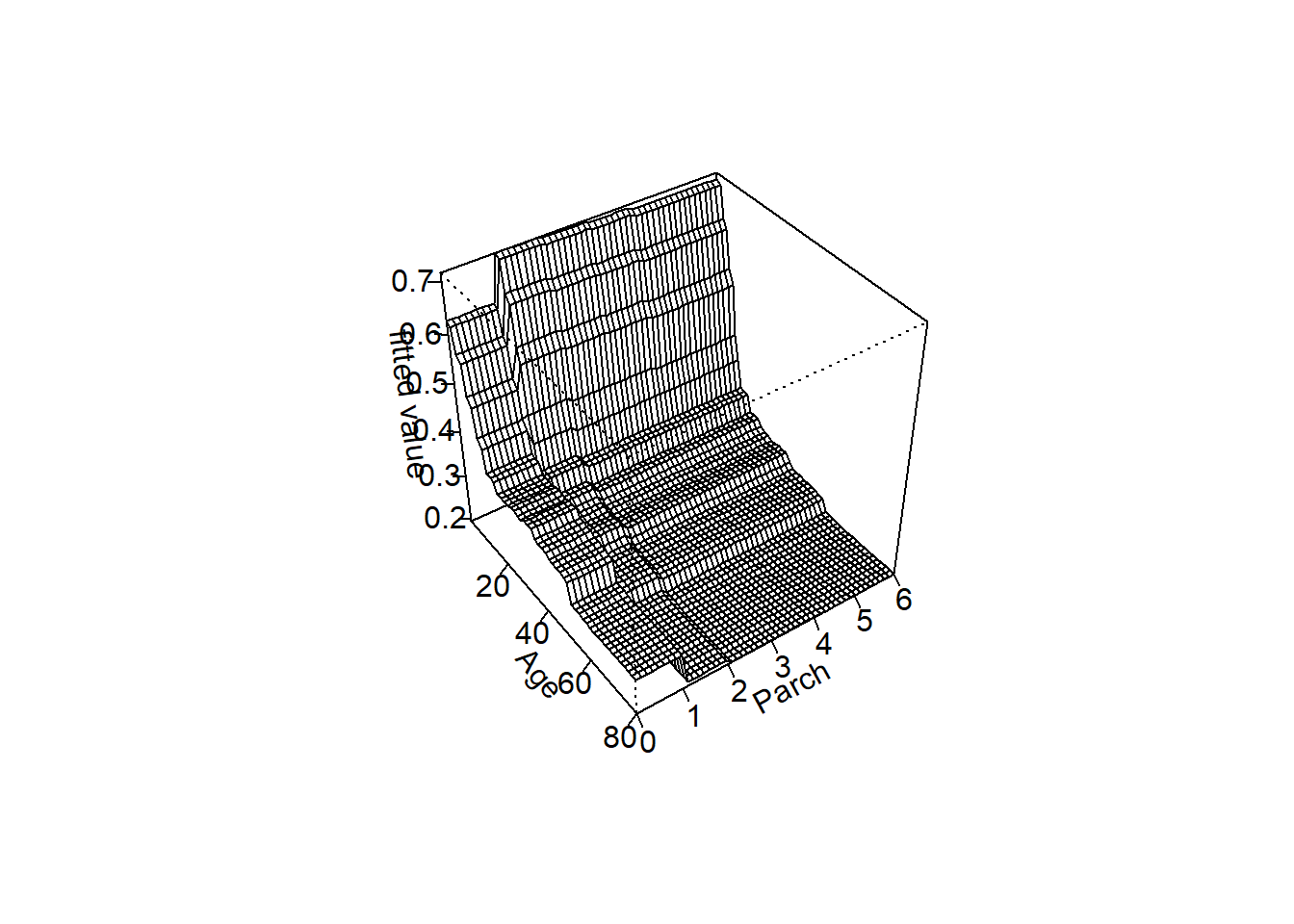## Model performance

Finally, let’s bring this home by looking at model performance!

###################################
#################### CROSS VALIDATION CODE

n.folds = 10       # set the number of "folds"
foldVector = rep(c(1:n.folds),times=floor(length(titanic$Survived)/9))[1:length(titanic$Survived)]

Then, we do the cross validation, looping through each fold of the data, leaving out each fold in turn for model training.

counter = 1
CV_df <- data.frame(
CVprediction = numeric(nrow(titanic)),      # make a data frame for storage
realprediction = 0,
realdata = 0
)

for(i in 1:n.folds){
fit_ndx <- which(foldVector!=i)
validate_ndx <- which(foldVector==i)
model <- cforest(formula1, data = titanic[fit_ndx,], controls=cforestControl)
predict_CV  <- predict(model,newdata=titanic[validate_ndx,],type="prob")
predict_real  <-  predict(rf_model1,newdata=titanic[validate_ndx,],type="prob")
REAL <- titanic$Survived[validate_ndx] for(j in 1:length(which(foldVector==i))){ CV_df$CVprediction[counter] <- as.numeric(predict_CV[[j]][,2])
CV_df$realprediction[counter] <- as.numeric(predict_real[[j]][,2]) CV_df$realdata[counter] <- REAL[j]
counter = counter + 1
}
}

fact=TRUE
if(fact){
CV_df$realdata=CV_df$realdata-1
}

CV_RMSE = sqrt(mean((CV_df$realdata - CV_df$CVprediction)^2))       # root mean squared error for holdout samples in 10-fold cross-validation
real_RMSE = sqrt(mean((CV_df$realdata - CV_df$realprediction)^2))  # root mean squared error for residuals from final model

# print RMSE statistics

cat("The RMSE for the model under cross-validation is: ", CV_RMSE, "\n")
## The RMSE for the model under cross-validation is:  0.3748092
cat("The RMSE for the model using all data for training is: ", real_RMSE, "\n")
## The RMSE for the model using all data for training is:  0.3547742

Let’s plot out the ROC curves!

library(ROCR)
library(rms)

par(mfrow=c(2,1))
pred <- prediction(CV_df$CVprediction,CV_df$realdata)     # for holdout samples in cross-validation
perf <- performance(pred,"tpr","fpr")
auc <- performance(pred,"auc")
plot(perf, main="Cross-validation")
text(.9,.1,paste("AUC = ",round(auc@y.values[],2),sep=""))

pred <- prediction(CV_df$realprediction,CV_df$realdata)     # for final model
perf <- performance(pred,"tpr","fpr")
auc <- performance(pred,"auc")
plot(perf, main="All data")
text(.9,.1,paste("AUC = ",round(auc@y.values[],2),sep=""))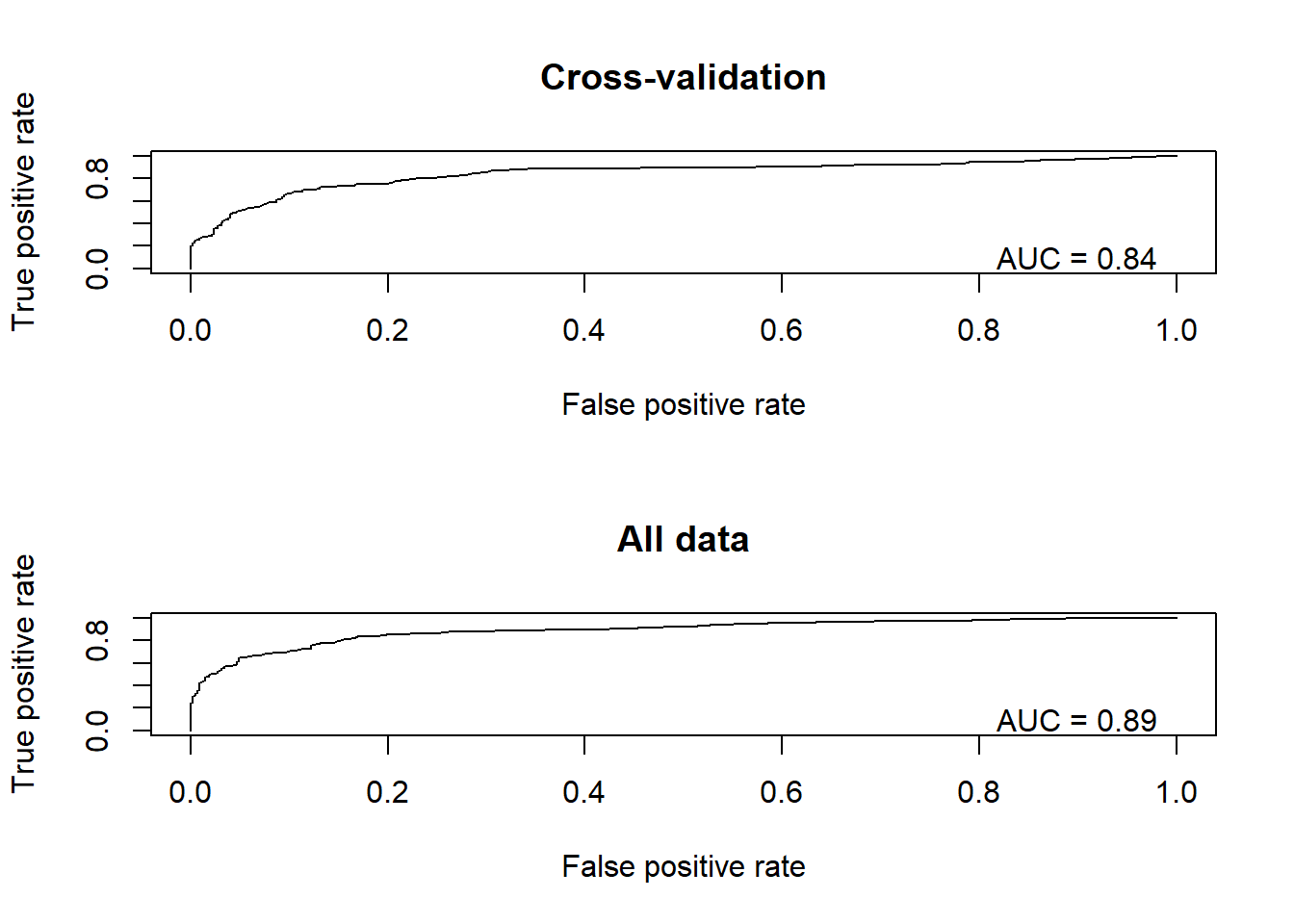Finally, we can use the same pseudo-R-squared metric we learned above as an alternative metric of performance

CV_df$CVprediction[which(CV_df$CVprediction==1)] <- 0.9999       # ensure that all predictions are not exactly 0 or 1
CV_df$CVprediction[which(CV_df$CVprediction==0)] <- 0.0001
CV_df$realprediction[which(CV_df$realprediction==1)] <- 0.9999
CV_df$realprediction[which(CV_df$realprediction==0)] <- 0.0001

fit_deviance_CV <- mean(-2*(dbinom(CV_df$realdata,1,CV_df$CVprediction,log=T)-dbinom(CV_df$realdata,1,CV_df$realdata,log=T)))
fit_deviance_real <- mean(-2*(dbinom(CV_df$realdata,1,CV_df$realprediction,log=T)-dbinom(CV_df$realdata,1,CV_df$realdata,log=T)))
null_deviance <- mean(-2*(dbinom(CV_df$realdata,1,mean(CV_df$realdata),log=T)-dbinom(CV_df$realdata,1,CV_df$realdata,log=T)))
deviance_explained_CV <- (null_deviance-fit_deviance_CV)/null_deviance   # based on holdout samples
deviance_explained_real <- (null_deviance-fit_deviance_real)/null_deviance   # based on full model...

# print RMSE statistics

cat("The McFadden R2 for the model under cross-validation is: ", deviance_explained_CV, "\n")
## The McFadden R2 for the model under cross-validation is:  0.3355542
cat("The McFadden R2 for the model using all data for training is: ", deviance_explained_real, "\n")
## The McFadden R2 for the model using all data for training is:  0.3922678

[–this is the final regular lecture–]